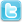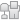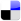Samakomlao -> Samakomlao Main -> Lao girls Zone -> God and super natural power .
Post Info TOPIC: God and super natural power .Guru

Status: Offline
Posts: 693
Date:
 God and super natural power . Permalink If God is delusion which separated and created war among different cultures and different races .Now science could prove that God didn't create the universe and there are no super natural power in the universe and no reincarnation and no life after death that means when you are dead , you are finished. There are more men killing each other over my God is better than your God for thousand of years. Will there still be Holy war and suicide Bombers (No more 40 virgins are waiting for them in heaven ) since Osama  Bin Laden was killed and the US Navy Seals proved that Osama Bin Laden was not under the protection of Alla ( God ). But the scientists (Cern) will be able to prove that how the universe began from the Big Bang. Could human being live in peace  without God or religions ?-- Edited by Dark Angel on Saturday 7th of May 2011 08:23:51 AM __________________

Senior Member

Status: Offline
Posts: 194
Date:
 Permalink Do you Beleive in God  Dark Angel? __________________
Anonymous

Date:
 Permalink taxi wrote:Do you Beleive in God  Dark Angel? I believe in law of nature and law of physics, right and wrong and good and evil . I don't believe in personal God who created the universe in 7 days and the world is only 6000 years old. __________________Guru

Status: Offline
Posts: 693
Date:
 Permalink taxi wrote:Do you Beleive in God  Dark Angel?     Hell no. I believe in law of nature and law of physics and science . __________________
Anonymous

Date:
 Permalink Dark Angel wrote:taxi wrote:Do you Beleive in God  Dark Angel?     Hell no. I believe in law of nature and law of physics and science . Umm sound similar to Buddha's teaching do you think so, Dark Angel? __________________Guru

Status: Offline
Posts: 693
Date:

Equation of state (cosmology)

Physical cosmologyUniverse · Big Bang
Age of the universe
Timeline of the Big Bang
Ultimate fate of the universe
v · d · e

In cosmology, the equation of state of a perfect fluid is characterized by a dimensionless number$\! w$, equal to the ratio of its pressure$\! p$ to its energy density$\!
ho$
:$\! w=p/
ho$
. It is closely related to the thermodynamic equation of state and ideal gas law.

[hide]

The equation

The perfect gas equation of state may be written as$\! p =
ho_m RT =
ho_m C^2$

where$\!
ho_m$
is the mass density,$\! R$ is the particular gas constant,$\! T$ is the temperature and$\! C=\sqrt{RT}$ is a characteristic thermal speed of the molecules. Thus$w = \frac{p}{
ho} = \frac{
ho_mC^2}{
ho_mc^2} = \frac{C^2}{c^2}\approx 0$

where$\!
ho =
ho_mc^2$
and$\! C< for a "cold" gas,$\! c$ - speed of light.

FLRW equations and the equation of state

The equation of state may be used in Friedmann-Lemaître-Robertson-Walker equations to describe the evolution of an isotropic universe filled with a perfect fluid. If$\! a$ is the scale factor then$
ho\propto a^{-3(1+w)}.$

If the fluid is the dominant form of matter in a flat universe, then$a\propto t^{\frac{2}{3(1+w)}},$

where$\! t$ is the proper time.

In general the Friedmann acceleration equation is$3\frac{\ddot{a}}{a} = \Lambda - 4 \pi G (
ho + 3p)$

where$\! \Lambda$ is the cosmological constant and$\! G$ is Newton's constant, and$\ddot{a}$ is the second proper time derivative of the scale factor.

If we define (what might be called "effective") energy density and pressure as$
ho^\prime \equiv
ho + \frac{\Lambda}{8 \pi G}$$p^\prime \equiv p - \frac{\Lambda}{8 \pi G}$

and$p^\prime = w^\prime
ho^\prime$

the acceleration equation may be written as$\frac{\ddot a}{a}=-\frac{4}{3}\pi G\left(
ho^\prime + 3p^\prime
ight) = -\frac{4}{3}\pi G(1+3w^\prime)
ho^\prime$

Non-relativistic matter

The equation of state of ordinary non-relativistic matter (e.g. cold dust) is$\! w=0$, which means that it is diluted as$
ho\propto a^{-3}=V^{-1}$
, where$\! V$ is the volume. This means that the energy density red-shifts as the volume, which is natural for ordinary non-relativistic matter.

Ultra-relativistic matter

The equation of state of ultra-relativistic matter (e.g. radiation, but also matter in the very early universe) is$\! w=1/3$ which means that it is diluted as$
ho\propto a^{-4}$
. In an expanding universe, the energy density decreases more quickly than the volume expansion, because radiation has momentum and, by the de Broglie hypothesis a wavelength, which is red-shifted.

Acceleration of cosmic inflation

Cosmic inflation and the accelerated expansion of the universe can be characterized by the equation of state of dark energy. In the simplest case, the equation of state of the cosmological constant is$\! w=-1$. In this case, the above expression for the scale factor is not valid and$a\propto e^{Ht}$, where the constant H is the Hubble parameter. More generally, the expansion of the universe is accelerating for any equation of state$\! w<-1/3$. The accelerated expansion of the Universe was indeed observed. According to observations, the value of equation of state of cosmological constant is near -1.

Hypothetical phantom energy would have an equation of state$\! w<-1$, and would cause a Big Rip. Using the existing data, it is still impossible to distinguish between phantom$\! w<-1$ and non-phantom$\! w\ge-1$.

Fluids

In an expanding universe, fluids with larger equations of state disappear more quickly than those with smaller equations of state. This is the origin of the flatness and monopole problems of the big bang: curvature has$\! w=-1/3$ and monopoles have$\! w=0$, so if they were around at the time of the early big bang, they should still be visible today. These problems are solved by cosmic inflation which has$\! w\approx -1$. Measuring the equation of state of dark energy is one of the largest efforts of observational cosmology. By accurately measuring$\! w$, it is hoped that the cosmological constant could be distinguished from quintessence which has$\! w\ne -1$.

Scalar modeling

A scalar field$\! \phi$ can be viewed as a sort of perfect fluid with equation of state${w=\frac{\frac{1}{2}\dot{\phi}^2-V(\phi)}{\frac{1}{2}\dot{\phi}^2+V(\phi)},}$

where$\! \dot{\phi}$ is the time-derivative of$\! \phi$ and$\! V(\phi)$ is the potential energy. A free$\! (V=0)$ scalar field has$\! w=1$, and one with vanishing kinetic energy is equivalent to a cosmological constant:$\! w=-1$. Any equation of state in between, but not crossing the$\! w=-1$ barrier known as the Phantom Divide Line (PDL), is achievable, which makes scalar fields useful models for many phenomena in cosmology.

Notes

1. ^ Hogan, Jenny. "Welcome to the Dark Side." Nature 448.7151 (2007): 240-245. http://www.nature.com/nature/journal/v448/n7151/full/448240a.html
2. ^ A. Vikman,Can dark energy evolve to the phantom?, Phys. Rev. D 71, 023515 (2005), http://www.slac.stanford.edu/spires/find/hep/www?rawcmd=bb+astro-ph%2F0407107&FORMAT=WWW&SEQUENCE=

__________________
Anonymous

Date:
Anonymous

Date:

Veteran Member

Status: Offline
Posts: 52
Date:
 Permalink Anonymous wrote:Dark Angel wrote:taxi wrote:Do you Beleive in God  Dark Angel?     Hell no. I believe in law of nature and law of physics and science . Umm sound similar to Buddha's teaching do you think so, Dark Angel?  1.  I think he believes not getting down on your knees and kissing some guys foot and feeding him.  Then he scams you.2.  Dying to just to get 30 virgins, when u are dead.  Well u can't do them, they have to stay virgins, so u can only look not touch.  Bunch of Morons.3.  Throwing money away to the white man, that doesn't exist.  If I throw money in the sky and if it don't come down, he can have it. If you want to know the truth about religions, what isn't taught in School and other places.  It's ment to control the people and Taxes.  But many use it for comfort when they are about to die. __________________
 Page 1 of 1  sorted by Oldest FirstNewest First